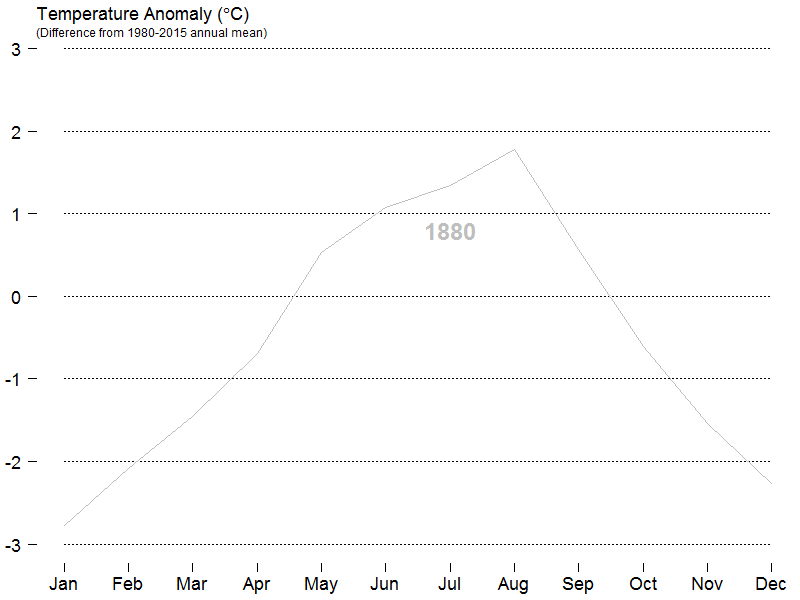# How to produce an animated gif of rise in global temperature in R

September 15, 2016
By

Want to share your content on R-bloggers? click here if you have a blog, or here if you don't.

I came across a really cool tweet a few days ago containing an animated GIF demonstrating rise in global temperatures since 1880:

Link to the blog is here.

I wanted to see if I could recreate this GIF in R, and below are some instructions on how to get close.

The first step is to try and see if I can replicate the original, final image.  The code below comes close enough for this demo, but strangely I am approx. 1 degree C out.  I’ve left a comment on the original blog to ask why – it’s not clear how the exact figures are calculated.

Anyway, here is how to get close:

``````
skip=7, header=TRUE, nrows=143, colClasses = "character")

# clean data
d <- d[-grep("Year", d\$Year),]
rownames(d) <- d[,1]
d <- d[,-1]
d <- d[,-13:-19]
d[d=="****"] <- NA

# convert to numeric and celsius
for (i in 1:12) {
d[,i] <- as.numeric(d[,i]) / 100
}

sa <- as.numeric(s\$seas_anom)

# create colours from blue through to red
colours <- colorRampPalette(c("grey","blue","red"))(nrow(d))

# create initial plot
mmin <- -3
mmax <- 3
par(mar=c(2,2,2,0))
plot(1:12, d[1,]+sa, type="l", ylim=c(mmin,mmax),
yaxt="n", xaxt="n", bty="n")

axis(side=1, at=1:12,
labels=c("Jan","Feb","Mar",
"Apr","May","Jun",
"Jul","Aug","Sep",
"Oct","Nov","Dec"),
lwd=0, lwd.ticks=1, cex.axis=0.8)

axis(side=2, at=-3:3, labels=-3:3,
lwd=0, lwd.ticks=1, las=2, cex.axis=0.8)

title(main=expression(paste("Temperature ","Anomaly ","(",degree,"C)")),
title(main="(Difference from 1980-2015 annual mean)", adj=0, cex.main=0.6,
line=0, font.main=1)

for (j in -3:3) {
lines(1:12, rep(j, 12), lty=3)
}

for (j in 1:nrow(d)) {
lines(1:12, as.numeric(d[j,])+sa, col=colours[j])
}

``````

This produces the following image:This is close enough!

So how do we create the animation?  The old skool way of doing this is to create every image in R and create an animated GIF with ImageMagick.  So we'll do thatThe slightly adjusted code to create every image is here (creates 137 PNGs in working directory!):

``````
#
# Assumes you have run the code above to create d and sa
#

doplot <- function(i) {

x   <- d[i,] + sa
col <- colours[i]

mmin <- -3
mmax <- 3
par(mar=c(2.5,2.5,2,0))
plot(1:12, x, type="l", ylim=c(mmin,mmax),
yaxt="n", xaxt="n", bty="n", col=col)
axis(side=1, at=1:12, labels=c("Jan","Feb","Mar",
"Apr","May","Jun",
"Jul","Aug","Sep",
"Oct","Nov","Dec"),
lwd=0, lwd.ticks=1, cex.axis=1.5)

axis(side=2, at=-3:3, labels=-3:3, lwd=0,
lwd.ticks=1, las=2, cex.axis=1.5)

title(main=expression(paste("Temperature ","Anomaly ","(",degree,"C)")),
title(main="(Difference from 1980-2015 annual mean)",

for (j in -3:3) {
lines(1:12, rep(j, 12), lty=3)
}

for (k in 1:i) {
lines(1:12, d[k,]+sa, col=colours[k])
}

text(7, 0.8, rownames(d)[i], col=colours[i], font=2, cex=2)

}

# create plots/PNGs
for (j in 1:nrow(d)) {

name <- paste("plot",sprintf("%03.f", j),".png", sep="")
png(name, width=800, height=600)
doplot(j)
dev.off()
}
``````

Then in ImageMagick, we use convert to create the animated GIF:

``````
convert *.png -delay 3 -loop 1 globaltemp.gif
convert globaltemp.gif \( +clone -set delay 500 \) +swap +delete globaltempwpause.gif
```
```

Final result here:DoneR-bloggers.com offers daily e-mail updates about R news and tutorials about learning R and many other topics. Click here if you're looking to post or find an R/data-science job.
Want to share your content on R-bloggers? click here if you have a blog, or here if you don't.